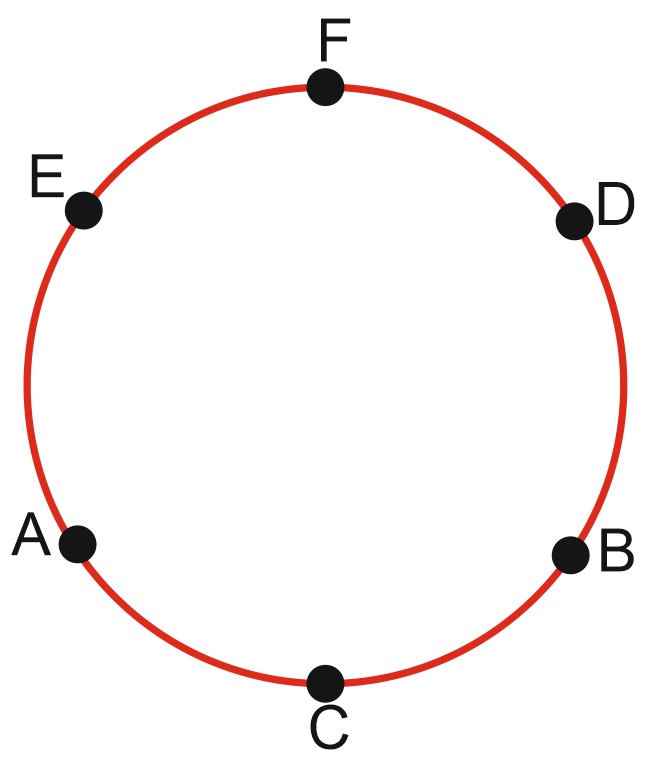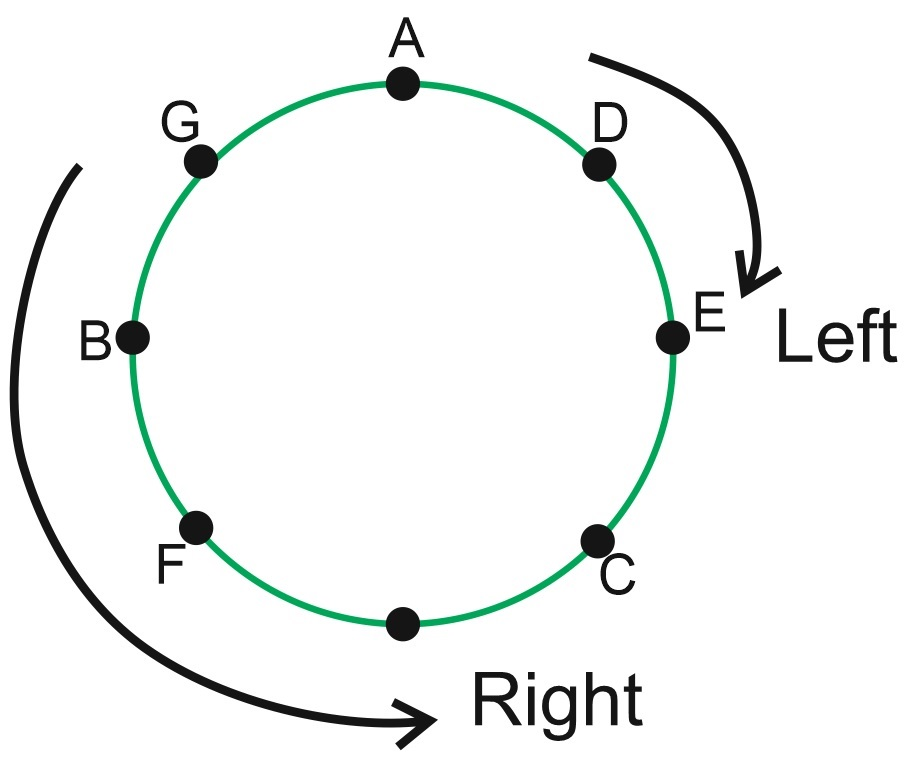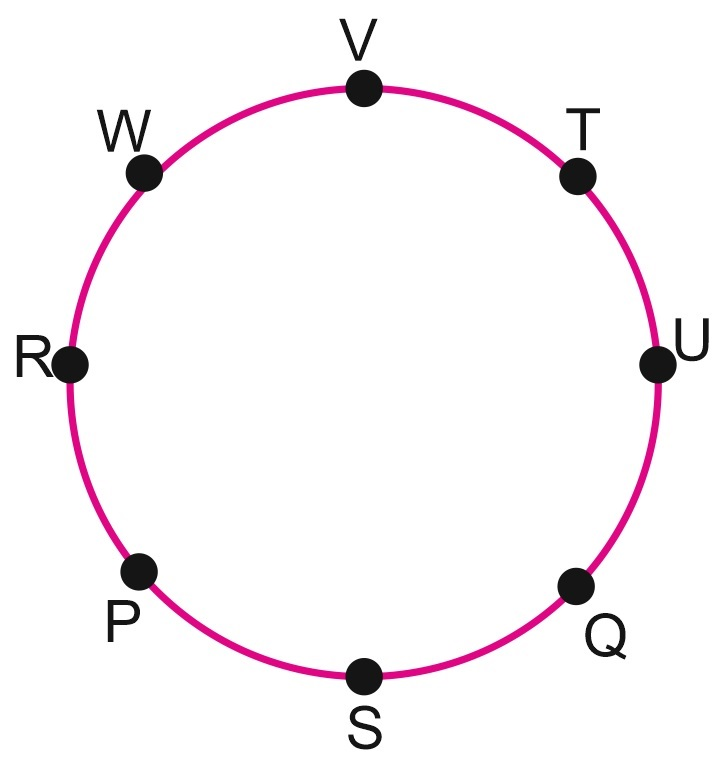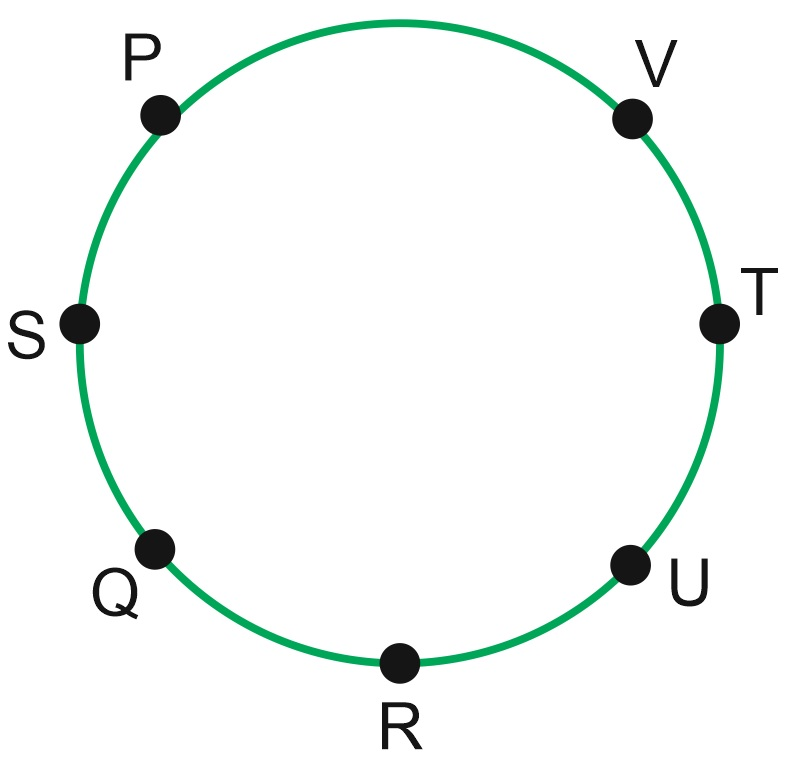• Save

# Seating Arrangement Problems with Solutions for SSC and Bank ExamsSeating arrangement questions are asked under the Reasoning section in SSC and Bank exams, which are about 3-4 questions in question paper. Also, if you practice this topic properly, you can easily get full marks in the exam. Therefore, with the help of the important questions provided here, solve these seating arrangement problems to increase your performance.

You can practice seating arrangement questions and answers also as well as circular seating arrangement questions for better performance. If you want to practice in Hindi visit here: seating arrangement questions in Hindi.

## Seating Arrangement Problems with Solutions for Competitive Exams

Q.1. Six persons A, B, C, D, E & F are standing in a circle. B is between D & C. A is between E & C. F is at the right of D. Who is between A & F?

(A) E

(B) C

(C) D

(D) None of these.

Ans (A)

SolutionE will be between A & F.

Q.2. A, B, C, D, E, F & G are sitting in a circular region & playing cards. F is 2nd to the right of G. B is neighbour of F but not of C. E is a neighbour of C & is siting 4th at the right of G. D is between E & A.

(1) Who is fourth at left of G ?

(A) D

(B) E

(C) C

(D) B

(2) Who is at left of G ?

(A) A

(B) C

(C) B

(D) F

(3) Who is the neighbour of F ?

(A) E and C

(B) F and B

(C) A and B

(D) C and B

Ans: (1) C, (2) A, (3)D

Solution

When the arrangement is given in circle, then there is a chance of mistake regarding left & right. So, we have to consider our self at the point of starting. Then think about your left & right. So, there will be no mistake.

If we start to solve the problem from left towards the downward, that will be more easier.Note – In this type of question, the word which or who is used for the II person & the words like but, while, whereas, and etc. are used for the I person.

Q.3. Eight friends – P, Q, R, S, T, U, V & W are sitting around a circle facing the center. V is third to the right of Q & second to the left of R. Q is second to the left of T & immediate right of S. U is between Q & T. P is not at the left of R.

(1) Who is exactly at left of S ?

(A) Q

(B) P

(C) U

(D) V

(2) Who is second to right of T ?

(A) R

(B) P

(C) W

(D) V

(3) In which of the following pairs first person sits exactly at right of second persons?

(A) UT

(B) WR

(C) WT

(D) VT

(4) What is the exact position of W?

(A) Just left of V

(B) Just right of V

(C) In between U and V

(D) Immediate right to R

(5) Who is second at right of S?

(A) U

(B) R

(C) T

(D) P

Ans : (1) B, (2)C , (3) D ,(4) B, (5) A

SolutionQ.4. (A) P, Q,R,S,T,U & V are sitting around a circle facing the center.

(B) P is between V and S.

(C) R, who is second to right of S, is between Q and U.

(D) Q is no the neighbour of T.

(1) Which of the following statement is true?

(A) V is between P and S.

(B) S is second to the left of V

(C) R is third to the left of P

(D) P is at immediate left of S

(2) What is the position of T?

(A) between R and V

(B) just left of V

(C) second to the left of R.

(D)second to the right of P

(3) Who is between R and U?

(A) none

(B) S

(C) V

(D) Q

(4) Which of the following statement is wrong?

(A) R is at immediate right of U.

(B) Q is at immediate left of R.

(C) T is third to the right of Q.

(D) U is third to the right of T.

(5) In which of the following pairs, the second member is at the immediate right of the first member?

(A) QS

(B) PV

(C) RU

(D) VT

Ans : (1) D, (2) B, (3) A, (4) A, (5) C

SolutionI hope these solutions are helpful for your preparation. You can ask me anything in the comment section related to seating arrangement problems with solutions if they face any problem or doubt.